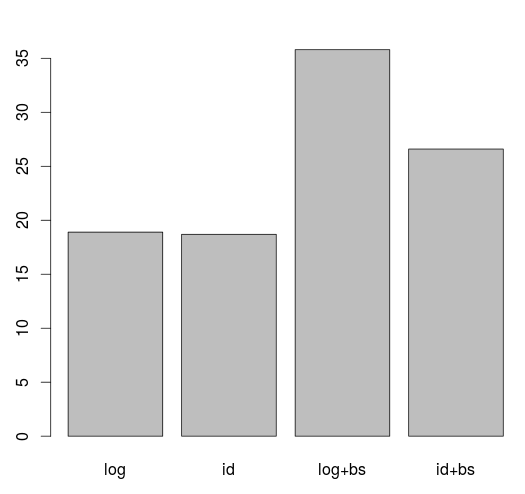Want to share your content on R-bloggers? click here if you have a blog, or here if you don't.

This afternoon, we’ve seen in the training on data science that it was possible to use AIC criteria for model selection.

```> library(splines)
> AIC(glm(dist ~ speed, data=train_cars,
 438.6314
> AIC(glm(dist ~ speed, data=train_cars,
 436.3997
> AIC(glm(dist ~ bs(speed), data=train_cars,
 425.6434
> AIC(glm(dist ~ bs(speed), data=train_cars,
 428.7195```

And I’ve been asked why we don’t use a training sample to fit a model, and then use a validation sample to compare predictive properties of those models, penalizing by the complexity of the model.    But it turns out that it is difficult to compute the AIC of those models on a different dataset. I mean, it is possible to write down the likelihood (since we have a Poisson model) but I want a code that could work for any model, any distribution….

Hopefully, Heather suggested a very clever idea, using her package

And actually, it works well.

Create here two datasets, one for the training, and one for validation

```> set.seed(1)
> idx = sample(1:50,size=10,replace=FALSE)
> train_cars = cars[-idx,]
> valid_cars = cars[idx,]```

then use simply

```> library(gnm)
> reg1 = gnm(dist ~ speed, data=train_cars,
> reg2 = gnm(dist ~ speed, data=valid_cars,
contrain = "*", contrainTo =
```

Here Akaike criteria on the validation sample is

```> AIC(reg2)
 82.57612```

Let us keep tracks of a prediction to plot it later on

```> v_log=predict(reg1,newdata=
data.frame(speed=u),type="response")```

We can challenge that Poisson model with a log link with a Poisson with a linear link function

```> reg1 = gnm(dist ~ speed, data=train_cars,
> reg2 = gnm(dist ~ speed, data=valid_cars,
contrain = "*", contrainTo =
> AIC(reg2)
 73.24745
> v_id=predict(reg1,newdata=data.frame(speed=u),
type="response")```

We can also try to include splines, either with the log link

```> library(splines)
> reg1 = gnm(dist ~ bs(speed), data=train_cars,
> reg2 = gnm(dist ~ speed, data=valid_cars,
contrain = "*", contrainTo =
> AIC(reg2)
 82.57612
> v_log_bs=predict(reg1,newdata=
data.frame(speed=u),type="response")```

or with the identity link (but always in the same family)

```> reg1 = gnm(dist ~ bs(speed), data=train_cars,
> reg2 = gnm(dist ~ speed, data=valid_cars,
contrain = "*", contrainTo =
> AIC(reg2)
 73.24745
> v_id_bs=predict(reg1,newdata=
data.frame(speed=u),type="response")```

If we plot the predictions, we get

```> plot(cars)
> points(train_cars,pch=19,cex=.85,col="grey")
> lines(u,v_log,col="blue")
> lines(u,v_id,col="red")
> lines(u,v_log_bs,col="blue",lty=2)
> lines(u,v_id_bs,col="red",lty=2)```Now, the problem with this holdout technique is that we might get unlucky (or lucky) when creating the samples. So why not try some monte carlo study, where many samples are generated,

```> four_aic=function(i){
+   idx = sample(1:50,size=10,replace=FALSE)
+   train_cars = cars[-idx,]
+   valid_cars = cars[idx,]
+   reg1 = gnm(dist ~ speed, data=train_cars,
+   reg2 = gnm(dist ~ speed, data=valid_cars,
contrain = "*", contrainTo =
+   a1=AIC(reg2)
+   reg0 = lm(dist ~ speed, data=train_cars)
+   reg1 = gnm(dist ~ speed, data=train_cars,
start=c(1,1))
+   reg2 = gnm(dist ~ speed, data=valid_cars,
contrain = "*", contrainTo =
start=c(1,1))
+   a2=AIC(reg2)
+   reg1 = gnm(dist ~ bs(speed), data=train_cars,
+   reg2 = gnm(dist ~ bs(speed), data=valid_cars,
contrain = "*", contrainTo =
+   a3=AIC(reg2)
+   reg1 = gnm(dist ~ bs(speed), data=train_cars,
+   reg2 = gnm(dist ~ bs(speed), data=valid_cars,
contrain = "*", contrainTo =
+   a4=AIC(reg2)
+   return(c(a1,a2,a3,a4))}```

Consider for instance 1,000 scenarios

`> S = sapply(1:1000,four_aic)`

The model that has, on average, the lowest AIC on a validation sample was the log-link with splines

```> rownames(S) = c("log","id","log+bs","id+bs")
> W = apply(S,2,which.min)
> barplot(table(W)/10,names.arg=rownames(S))```And indeed,

`> boxplot(t(S))`with that model, the AIC is usually lower with the spline model with a log link than the other one (or at least almost the same as the spline model with an identity link). Or at least, we can confirm here that a nonlinear model should be better than a nonlinear one.